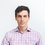# Simple arguments for mass energy equivalenceReading Steven Zheng's note on manipulating some of the equations of special relativity, I wondered how enlightening the calculations would be for someone who' d never studied relativity before. Therefore, I want to collect some simple, if not completely rigorous, arguments for mass-energy equivalence that make it clear why physics should have a relation between mass and energy. I'll make my own contributions in a few days and will select the clearest demonstrations from the comments. The connection between mass and energy is by far the most well known result in physics and is deeply curious, its fame notwithstanding.

Photon ejection

By the mid-1800s, it was known that light has energy, and a momentum, related by $p = \frac{E}{c}$. This was established by two independent lines of argument, one being the Poynting vector that can be derived from Maxwell's equations, and the other being experiments that shone light on a vane of metal. With this connection, we can motivate the equivalence of mass and energy if we analyze a block that ejects a single photon.

Suppose that a block of mass $M$, sits at rest. Let us call the total energy of this state. Then, the block ejects a photon of energy $E_\gamma$, and momentum $p=E_\gamma/c$ to the right. For the time being, we write the mass of the block after ejecting the photon as $m$, not necessarily different from $M$. Due to momentum conservation, the block moves with velocity $p/m = E_\gamma/cm$ to the left.

Now, we look at this situation from the perspective of an observer who moves exactly downward at the speed $v$. Momentum in any direction is conserved before and after the photon ejection (regardless of frame), so we can write down $Mv = mv + E_\gamma^\prime/c\times \sin\theta$ (conservation of momentum in the $y$-direction). The photon's energy according to the moving observer is written as $E_\gamma^\prime$ to reflect the blueshift from the relative motion (see below).

From the photoelectric effect (known to be frequency dependent in 1902), we have $p=E_\gamma/c = hf/c$ in the original frame. To the observer, $f$ is shifted a la $\displaystyle f\rightarrow f\frac{c}{c-v_s}\approx f\left(1+v/c\right)$, so that $p^\prime_\gamma = p_\gamma \left(1+v/c\right)$. The momentum in the $y$-direction is then $p_\gamma^\prime \sin\theta$ which is $p_\gamma^\prime v/c = p_\gamma \left(1+v/c\right)v/c \approx p_\gamma v/c$ (since $v\ll c$).

Thus, conserving momentum in the $y$-direction, we have $Mv = mv + E_\gamma v/c^2$ or $\Delta M = \Delta E / c^2$. Incredibly, this is the condition for momentum conservation. The block's loss of mass upon photon ejection is inescapable as long as momentum is conserved. In other words, if we hadn't allowed for differing $M$, and $m$, we would have had to sacrifice the conservation of momentum.

Einstein's original derivation of $E=Mc^2$ involved the Lorentz transformations, and was rather needlessly complicated. This derivation is more in line with a version he published in 1945, long after his original works. It is interesting to realize that mass energy equivalence can be obtained, if a bit loosely, without the complex framework of special relativity.Note by Josh Silverman
6 years, 11 months ago

This discussion board is a place to discuss our Daily Challenges and the math and science related to those challenges. Explanations are more than just a solution — they should explain the steps and thinking strategies that you used to obtain the solution. Comments should further the discussion of math and science.

When posting on Brilliant:

• Use the emojis to react to an explanation, whether you're congratulating a job well done , or just really confused .
• Ask specific questions about the challenge or the steps in somebody's explanation. Well-posed questions can add a lot to the discussion, but posting "I don't understand!" doesn't help anyone.
• Try to contribute something new to the discussion, whether it is an extension, generalization or other idea related to the challenge.

MarkdownAppears as
*italics* or _italics_ italics
**bold** or __bold__ bold
- bulleted- list
• bulleted
• list
1. numbered2. list
1. numbered
2. list
Note: you must add a full line of space before and after lists for them to show up correctly
paragraph 1paragraph 2

paragraph 1

paragraph 2

[example link](https://brilliant.org)example link
> This is a quote
This is a quote
    # I indented these lines
# 4 spaces, and now they show
# up as a code block.

print "hello world"
# I indented these lines
# 4 spaces, and now they show
# up as a code block.

print "hello world"
MathAppears as
Remember to wrap math in $$ ... $$ or $ ... $ to ensure proper formatting.
2 \times 3 $2 \times 3$
2^{34} $2^{34}$
a_{i-1} $a_{i-1}$
\frac{2}{3} $\frac{2}{3}$
\sqrt{2} $\sqrt{2}$
\sum_{i=1}^3 $\sum_{i=1}^3$
\sin \theta $\sin \theta$
\boxed{123} $\boxed{123}$

Sort by:

How is the picture related to this?

- 6 years, 11 months ago

It's the cover of this relativity book: Einstein Gravity in a Nutshell

Staff - 6 years, 11 months ago

I wrote a new note on that includes the derivation of relativistic energy from first principles (make special relativity work under basic Newtonian definitions). Check it out here Discussion: Why E = mc2

However, I would say it is rather reductionist. So if you want to explore more of the why? I'd be interested to see what you can find.

- 6 years, 11 months ago

Yes, it is fine given $p=\gamma m v$ with the form for $\gamma$ and all that. But that formula isn't known by someone who hasn't studied relativity. I'm thinking of arguments to motivate the mass energy equivalence without assuming special relativity.

Staff - 6 years, 11 months ago